#### Exact solutions

Suppose that all edges in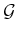are line segments in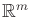for some dimension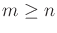. An edge that is generated early in the construction process will be split many times in later iterations. For the purposes of finding the nearest point in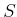, however, it is best to handle this as a single segment. For example, see the three large branches that extend from the root in Figure 5.19. As the number of points increases, the benefit of agglomerating the segments increases. Let each of these agglomerated segments be referred to as a supersegment. To implement NEAREST, a primitive is needed that computes the distance between a point and a line segment. This can be performed in constant time with simple vector calculus. Using this primitive, NEAREST is implemented by iterating over all supersegments and taking the point with minimum distance among all of them. It may be possible to improve performance by building hierarchical data structures that can eliminate large sets of supersegments, but this remains to be seen experimentally.

In some cases, the edges ofmay not be line segments. For example, the shortest paths between two points in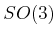are actually circular arcs along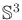. One possible solution is to maintain a separate parameterization offor the purposes of computing the NEAREST function. For example,can be represented as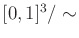, by making the appropriate identifications to obtain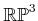. Straight-line segments can then be used. The problem is that the resulting metric is not consistent with the Haar measure, which means that an accidental bias would result. Another option is to tightly enclosein a 4D cube. Every point oncan be mapped outward onto a cube face. Due to antipodal identification, only four of the eight cube faces need to be used to obtain a bijection between the set of all rotation and the cube surface. Linear interpolation can be used along the cube faces, as long as both points remain on the same face. If the points are on different faces, then two line segments can be used by bending the shortest path around the corner between the two faces. This scheme will result in less distortion than mappingto; however, some distortion will still exist.

Another approach is to avoid distortion altogether and implement primitives that can compute the distance between a point and a curve. In the case of, a primitive is needed that can find the distance between a circular arc inand a point in. This might not be too difficult, but if the curves are more complicated, then an exact implementation of the NEAREST function may be too expensive computationally.

Steven M LaValle 2020-08-14